# Using division of polynomials, state whether`
Question:

Using division of polynomials, state whether

(i) x + 6 is a factor of  x2 − x − 42

(ii) 4x − 1 is a factor of 4x2 − 13x − 12

(iii) 2y − 5 is a factor of 4y4 − 10y3 − 10y2 + 30y − 15

(iv) 3y2 + 5 is a factor of 6y5 + 15y4 + 16y3 + 4y2 + 10y − 35

(v) z2 + 3 is a factor of z5 − 9z

(vi) 2x2 − x + 3 is a factor of 6x5 − x4 + 4x3 − 5x2 − x − 15

Solution:

(i)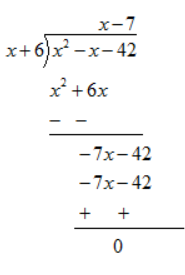Remainder is zero. Hence (x+6) is a factor of x2 -x-42

(ii)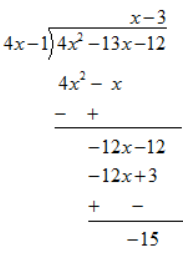As the remainder is non zero . Hence ( 4x-1) is not a factor of 4x2 -13x-12

(iii)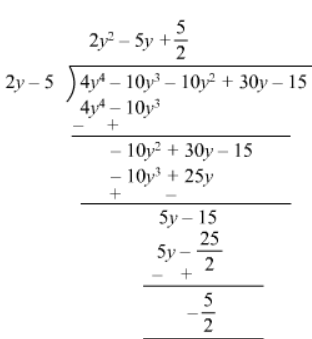$\because$ The remainder is non zero,

$2 y-5$ is not a factor of $4 y^{4}-10 y^{3}-10 y^{2}+30 y-15$.

(iv)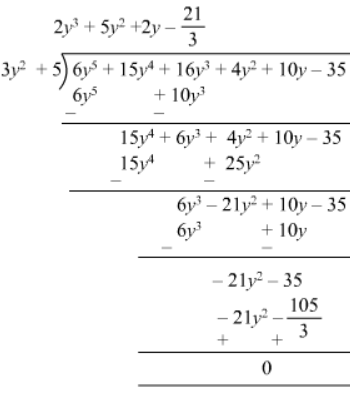Remainder is zero. Therefore, $3 y^{2}+5$ is a factor of $6 y^{5}+15 y^{4}+16 y^{3}+4 y^{2}+10 y-35$.

(v)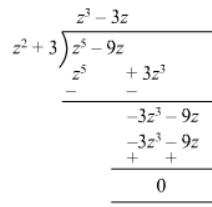Remainder is zero; therefore, $z^{2}+3$ is a factor of $z^{5}-9 z$.

(vi)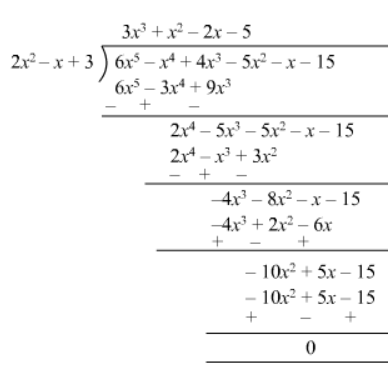Remainder is zero ; therefore, $2 x^{2}-x+3$ is a factor of $6 x^{5}-x^{4}+4 x^{3}-5 x^{2}-x-15$.